﻿ 长方体腔内关于密度极值温度对称加热-冷却时冷水瑞利-贝纳德对流稳定性
«上一篇文章快速检索 高级检索

 力学学报2015, Vol. 47Issue (5): 722-730  DOI: 10.6052/0459-1879-15-1710

### 引用本文 [复制中英文]

[复制中文]
Hu Yupeng, Li Yourong. NUMERICAL INVESTIGATION ON FLOW STABILITY OF RAYLEIGH-BĖNARD CONVECTION OF COLD WATER IN A RECTANGULAR CAVITY COOLED AND HEATED SYMMETRICALLY RELATIVE TO THE TEMPERATURE OF DENSITY MAXIMUM[J]. Chinese Journal of Ship Research, 2015, 47(5): 722-730. DOI: 10.6052/0459-1879-15-171.
[复制英文]

### 文章历史

2015-05-12收稿
2015-07-14录用
2015-07-20网络版发表

1. 中国工程物理研究院总体工程研究所, 绵阳621900;
2. 重庆大学动力工程学院, 重庆400044

|引 言

1 物理数学模型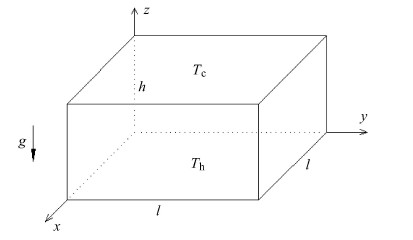图 1 物理模型 Fig.1 Physical model

 $\rho = {\rho _{\rm{m}}}{\rm{ }}\left( {1 - \gamma {{\left| {T - {T_{\rm{m}}}} \right|}^q}} \right)$ (1)

 $\nabla \cdot V = 0$ (2)
 ${\partial _\tau }V + V \cdot \nabla V = - \nabla P + {\nabla ^2}V + (Ra/Pr){\left| {\Theta - {\rm{ }}{\Theta _{\rm{m}}}} \right|^q}{e_Z}$ (3)
 ${\partial _\tau }{\rm{ }}\Theta + V \cdot \nabla {\rm{ }}\Theta = {\nabla ^2}\Theta /Pr$ (4)

 $N{u_{{\rm{ave}}}} = - \frac{1}{{{L^2}{\tau _{\rm{P}}}}}\int {_\tau ^{\tau + {\tau _{\rm{p}}}}} {\rm{ }}\int {_0^L} \int {_0^L} {\left| {\frac{{\partial \Theta }}{{\partial Z}}} \right|_{Z = 0}}{\rm{d}}X{\rm{d}}Yd\tau$ (5)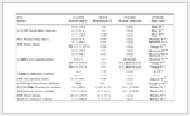表 1 常规流体瑞利-贝纳德对流壁面平均Nu对比 Table 1 Comparison of the average Nusselt number for R-B convection of common fluid
2 结果分析与讨论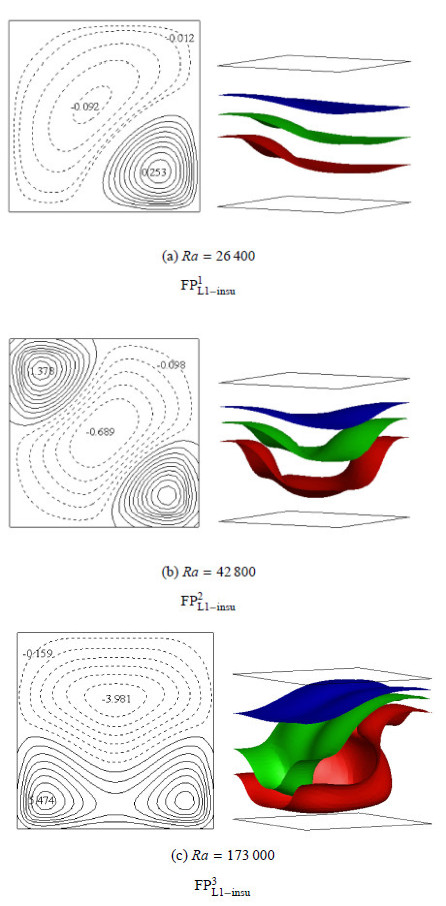图 2 L=1时绝热侧壁不同Ra下稳态流型. 左：Z=0.5截面等Z向速度线：实线为向上流动，速度为正， 虚线为向下流动，速度为负. 右：温度分别为$\Theta =0.3$(蓝)、0.5(绿)及0.7(红)对应的等温面(下同) Fig.2 Flow patterns for different Ra at L=1 for insulating sidewalls. left: contours of vertical velocity in the Z=0.5 plane. Solid lines denote a positive value and dotted lines denote a negative value. right: isothermal surfaces of $\Theta =0.3$ (blue), 0.5 (green) and 0.7 (red)(similarly hereinafter)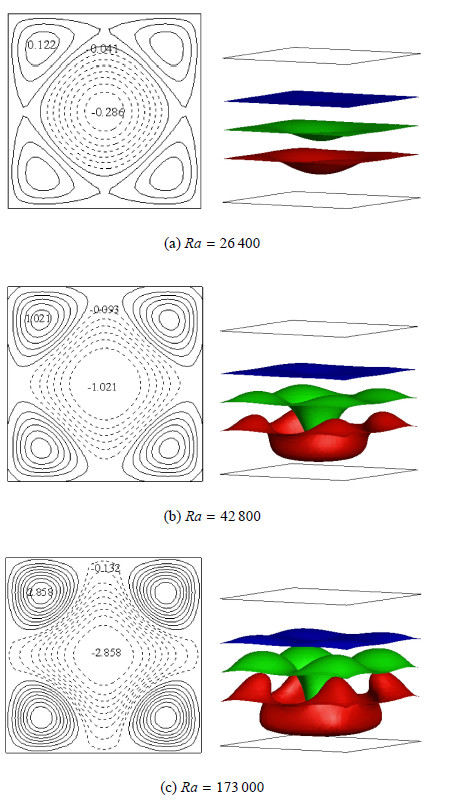图 3 L=1时导热侧壁不同$Ra$下稳态${\rm{FP}}_{{\rm{L1 - insu}}}^1$流型 Fig.3 Flow patterns of stable ${\rm{FP}}_{{\rm{L1 - insu}}}^1$ state at different $Ra$ for conducting sidewalls at L=1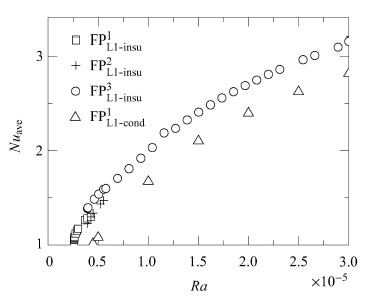图 4 L=1时热壁$Nu_{\rm ave}$随$Ra$的变化 Fig.4 The variation of the average Nusselt number at the hot wall at $L=1$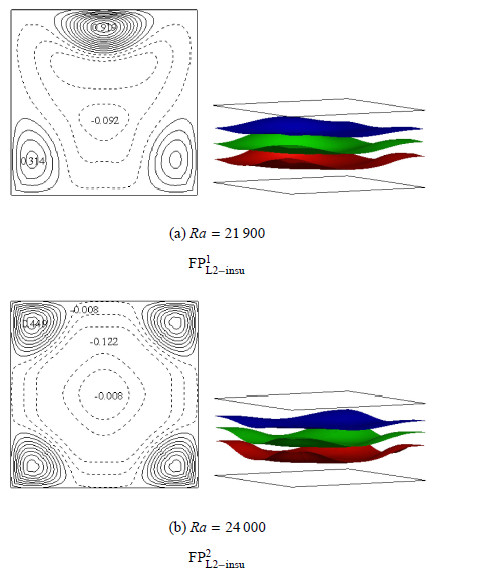图 5 $L =2$ 时绝热侧壁不同$Ra$下稳态流型 Fig.5 Flow patterns for different $Ra$ at $L =2$ for conducting sidewalls at $L =2$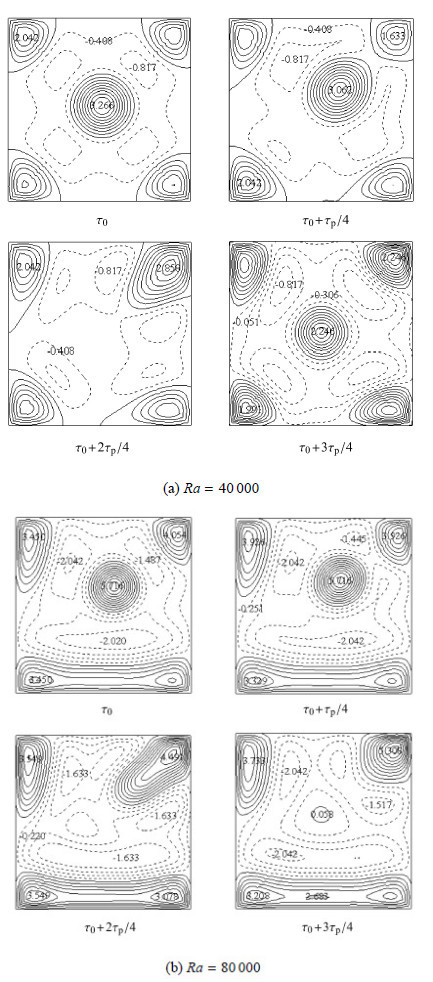图 6 $L =2$时一个周期内流型的演变过程 Fig.6 Flow evolution in a period at $L =2$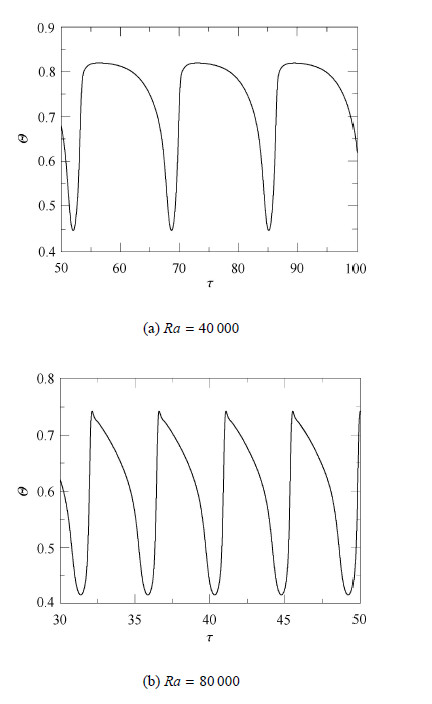图 7 $L =2$时监测点($X =0.5$，$Y =0.5$，$Z =0.5$)温度随时间演变 Fig.7 Time record of local temperature at a monitoring point ($X =0.5$，$Y =0.5$，$Z =0.5$) at $L =2$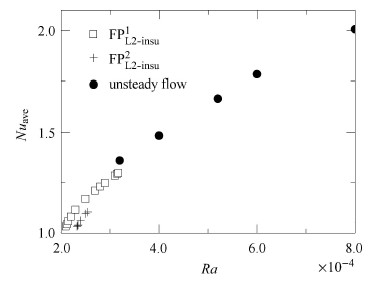图 8 $L =2$时热壁 $Nu_{\rm ave}$随$Ra$的变化 Fig.8 The variation of the average Nusselt number at the hot wall at $L=2$表 2 长方体腔热壁$Nu_{\rm ave}$关联式 Table 2 Correlations of the average Nusselt number on the hot wall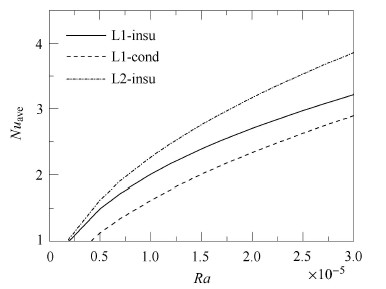图 9 不同条件下壁面传热能力比较 Fig.9 Comparison of the heat transfer ability for different conditions
3 结 论

  Cross MC, Hohenberg PC. Pattern formation outside of equilibrium. Reviews of Modern Physics, 1993, 65: 851-1086  Padilla ELM, Lourenço MAS, Silveira-Neto A. Natural convection inside cubical cavities: numerical solutions with two boundary conditions. Journal of the Brazilian Society of Mechanical Sciences and Engineering, 2013, 35(3): 275-283  王小华, 朱文芳. 长方腔自然对流第一次分岔突变现象的数值分析. 力学学报, 2010, 43(3): 389-399 (Wang Xiaohua, Zhu Wenfang. Numerical research on the sudden change characteristic of the first bifurcation for natural convection of air enclosed in 2D rectangular cavity. Chinese Journal of Theoretical and Applied Mechanics, 2010, 43(3): 389-399 (in Chinese))  孔祥言, 吴建兵. 多孔介质中的非达西自然对流的分岔研究. 力学学报, 2002, 34 (2): 177-185 (Kong Xiangyan, Wu Jianbing. A bifurcation study of non-Darcy free convection in porous media. Acta Mechanica Sinica, 2002, 34 (2): 177-185 (in Chinese))  Jeffreys H. Some cases of instability in fluid motion. Proceedings of the Royal Society of London Series A-Containing Papers of A Mathematical and Physical Character, 1928, 118(779): 195-208  Zhan NY, Xu PW, Sun SM, et al. Studu on the stability and 3-dimensional character for natural convection in a rectangular cavity heated from below. Science China Technological Sciences, 2010, 53(6): 1647-1654  Zhan NY, Gao Q, Bai L, et al. Experimental research on nonlinear characteristics of natural convection in a 3-D shallow cavity. Science China Technological Sciences, 2011, 54(12): 3304-3310  Mukutmoni D, Yang KT. Thermal convection in small enclosures: an atypical bifurcation sequence. International Journal of Heat and Mass Transfer, 1995, 38(1): 113-126  Gollub JP, Benson SV. Many routes to turbulent convection. Journal of Fluid Mechanics, 1980, 100(10): 449-470  Bousset F, Lyubimov DV, Sedel'Nikov GA. Three-dimensional convection regimes in a cubical cavity. Fluid Dynamics, 2008, 43(1): 1-8  Puigjaner D, Herrero J, Simó C, et al. Bifurcation analysis of steady Rayleigh-Bénard convection in a cubical cavity with conducting sidewalls. Journal of Fluid Mechanics, 2008, 598: 393-427  卞恩杰, 杨茉, 李凌等. 格子Boltzmann方法对Rayleigh-Bénard流的模拟与非线性分析. 工程热物理学报, 2012, 33(4): 685-688 (Bian Enjie, Yang Mo, Li Ling, et al. Simulation and nonlinear analysis for the Rayleigh-Bénard convection with the lattice Boltzmann method. Journal of Engineering Thermophysics, 2012, 33(4): 685-688 (in Chinese))  Leong SS. Numerical study of Rayleigh-Bénard convection in a cylinder. Numerical Heat Transfer Part A-Applications, 2002, 41: 673-683  Venturi D, Wan X, Karniadakis GE. Stochastic bifurcation analysis of Rayleigh-Bénard convection. Journal of Fluid Mechanics, 2010, 650(1): 391-413  Hof B, Lucas P, Mullin T. Flow state multiplicity in convection. Physics of Fluids, 1999, 11(10): 2815-2817  Borońska K, Tuckerman LS. Extreme multiplicity in cylindrical Rayleigh-Bénard convection. I. Time dependence and oscillations. Physical Review E, 2010, 81: 036320  Borońska K, Tuckerman LS. Extreme multiplicity in cylindrical Rayleigh-Bénard convection. II. Bifurcation diagram and symmetry classification. Physical Review E, 2010, 81: 036321  宁利中, 齐昕, 余荔等. 混合流体Rayleigh-Bénard对流的多重稳定性现象. 应用基础与工程科学学报, 2010, 18(2): 281-290 (Ning Lizhong, Qi Xing, Yu Li, et al. Multistability of Rayleigh-Bénard convection in a binary fluid mixture. Journal of Basic Science and Engineering, 2010, 18(2): 281-290 (in Chinese))  苏燕兵, 陆军, 白博峰. 封闭腔内水自然对流换热数值模拟. 化工学报, 2007, 58(11): 2715-2720 (Su Yanbing, Lu Jun, Bai Bofeng. Numerical simulation of natural convection and heat transfer of water in cavities. Journal of Chemical Industry and Engineering (China), 2007, 58(11): 2715-2720 (in Chinese))  Sivasankaran S, Ho CJ. Effect of temperature dependent properties on natural convection of water near its density maximum in enclosures. Numerical Heat Transfer Part A-Applications, 2008, 53: 507-523  Kashani S, Ranjbar AA, Mastiani M, et al. Entropy generation and natural convection of nanoparticle-water mixture (nanofluid) near water density inversion in an enclosure with various patterns of vertical wavy walls. Applied Mathematics and Computation, 2014, 226: 180-193  Braga SL, Viskanta R. Effect of density extremum on the solidification of water on a vertical wall of a rectangular cavity. Experimental Thermal and Fluid Science, 1992, 5(6): 703-713  Kandaswarmy P, Sivasankaran S, Nithyadevi N. Buoyancy-driven convection of water near its density maximum with partially active vertical walls. International Journal of Heat and Mass Transfer, 2007, 50: 942-948  Nithyadevi N, Sivasankaran S, Kandaswamry P. Buoyancy-driven convection of water near its density maximum with time periodic partially active vertical walls. Meccanica, 2007, 42: 503-510  Hu YP, Li YR, Yuan XF, et al. Natural convection of cold water near its density maximum in an elliptical enclosure containing a coaxial cylinder. International Journal of Heat and Mass Transfer, 2013, 60: 170-179  Hu YP, Li YR, Wu CM. Comparison investigation on natural convection of cold water near its density maximum in annular enclosures with complex configurations. International Journal of Heat and Mass Transfer, 2014, 72: 572-584  Li YR, Ouyang YQ, Hu YP. Pattern formation of Rayleigh-Bénard convection of cold water near its density maximum in a vertical cylindrical container. Physical Review E, 2012, 86: 046323  Gebhart B, Mollendorf JC. A new density relation for pure and saline water. Deep-Sea Research, 1977, 24: 831-848
NUMERICAL INVESTIGATION ON FLOW STABILITY OF RAYLEIGH-BĖNARD CONVECTION OF COLD WATER IN A RECTANGULAR CAVITY COOLED AND HEATED SYMMETRICALLY RELATIVE TO THE TEMPERATURE OF DENSITY MAXIMUM
1. Institute of systems Engineering, China Academy of Engineering Physics, Mianyang 621900, China;
2. College of Power Engineering, Chongqing University, Chongqing 400044, China
Fund: The project was supported by the National Natural Science Foundation of China (51376199) and the Innovation and Developing Foundation of ISE.CAEP (14cxj20).
Abstract: In order to understand the special phenomena and laws of Rayleigh-Bénard convection of fluids with density extremum, a series of three-dimensional numerical simulations on Rayleigh-Bénard convection of cold water in a rectangular cavity when its horizontal walls were cooled and heated symmetrically relative to the temperature of the density extremum by using finite volume method is carried out. Flow structures and their bifurcation series are obtained, and the effects of the density extremum character, the Rayleigh number, the thermal boundary condition and the aspect ratio on Rayleigh-Bénard convection are discussed. The results demonstrate that the system of Rayleigh-Bénard convection of cold water with density extremum is much more stable than that of common fluid, and the flow structures and their bifurcation series are much more complex. Multiple flow patterns can coexist at a constant Rayleigh number and hysteresis phenomenon is observed in the flow evolution. The system loses its stability more easily and the heat transfer ability enhances with the increase of the aspect ratio. The system for conducting sidewalls is much more stable than that for insulating sidewalls and the heat transfer ability weakens. Furthermore, heat transfer correlations are proposed according to the multiple linear regression.
Key words: density extremum    Rayleigh-Bènard    nard convection    flow Patterns    bifurcation character    heat transfer